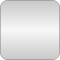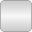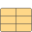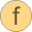# Common

Data structures and fundamental functions for fluid properties# Package Contents

 Rate (s-1) MolarFlowRate (mol/s) MolarReactionRate (mol/(m3.s)) MolarEnthalpy (J/mol) DerDensityByEntropy (kg2.K/(m3.J)) DerEnergyByPressure (J/Pa) DerEnergyByMoles (J/mol) DerEntropyByTemperature (J/K2) DerEntropyByPressure (J/(K.Pa)) DerEntropyByMoles (J/(mol.K)) DerPressureByDensity (Pa.m3/kg) DerPressureBySpecificVolume (Pa.kg/m3) DerPressureByTemperature (Pa/K) DerVolumeByTemperature (m3/K) DerVolumeByPressure (m3/Pa) DerVolumeByMoles (m3/mol) IsenthalpicExponent IsentropicExponent JouleThomsonCoefficient (K/Pa)ThermoFluidSpecial Property records used by the ThermoFluid librarySaturationProperties Properties in the two phase regionSaturationBoundaryProperties Properties on both phase boundaries, including some derivativesIF97BaseTwoPhase Intermediate property data record for IF 97IF97PhaseBoundaryProperties Thermodynamic base properties on the phase boundary for IF97 steam tablesGibbsDerivs Derivatives of dimensionless Gibbs-function w.r.t. dimensionless pressure and temperatureHelmholtzDerivs Derivatives of dimensionless Helmholtz-function w.r.t. dimensionless pressure, density and temperatureTwoPhaseTransportProps Defines properties on both phase boundaries, needed in the two phase regionPhaseBoundaryProperties Thermodynamic base properties on the phase boundaryNewtonDerivatives_ph Derivatives for fast inverse calculations of Helmholtz functions: p & hNewtonDerivatives_ps Derivatives for fast inverse calculation of Helmholtz functions: p & sNewtonDerivatives_pT Derivatives for fast inverse calculations of Helmholtz functions:p & TExtraDerivatives Additional thermodynamic derivativesBridgmansTables Calculates all entries in Bridgmans tables if first seven variables givenFundamentalConstants Constants of the mediumAuxiliaryProperties Intermediate property data recordGibbsDerivs2 Derivatives of Gibbs function w.r.t. pressure and temperatureNewtonDerivatives_dT Derivatives for fast inverse calculations of Gibbs functiongibbsToBridgmansTables Calculates base coefficients for Bridgman's tables from gibbs enthalpyhelmholtzToBridgmansTables Calculates base coefficients for Bridgmans tables from Helmholtz energygibbsToBoundaryProps Calculate phase boundary property record from dimensionless Gibbs functionhelmholtzToBoundaryProps Calculate phase boundary property record from dimensionless Helmholtz functioncv2Phase Compute isochoric specific heat capacity inside the two-phase regioncvdpT2Phase Compute isochoric specific heat capacity inside the two-phase region and derivative of pressure w.r.t. temperaturegibbsToExtraDerivs Compute additional thermodynamic derivatives from dimensionless Gibbs functionhelmholtzToExtraDerivs Compute additional thermodynamic derivatives from dimensionless Helmholtz functionHelmholtz_ph Function to calculate analytic derivatives for computing d and t given p and hHelmholtz_pT Function to calculate analytic derivatives for computing d and t given p and tHelmholtz_ps Function to calculate analytic derivatives for computing d and t given p and ssmoothStep Approximation of a general step, such that the characteristic is continuous and differentiableGibbs2_ph Function to calculate analytic derivatives for computing T given p and hGibbs2_dT Function to calculate analytic derivatives for computing p given d and TGibbs2_ps Function to calculate analytic derivatives for computing d and t given p and sOneNonLinearEquation Determine solution of a non-linear algebraic equation in one unknown without derivatives in a reliable and efficient way

# Package Constants (76)

MINPOS Value: 1.0e-9 Type: Real Description: Minimal value for physical variables which are always > 0.0 Value: MINPOS Type: Area (m²) Description: Minimal init area Value: 1.0e5 Type: Area (m²) Description: Maximal init area Value: 1.0 Type: Area (m²) Description: Nominal init area Value: -1.0 * MINPOS Type: AmountOfSubstance (mol) Description: Minimal Mole Number Value: 1.0e8 Type: AmountOfSubstance (mol) Description: Maximal Mole Number Value: 1.0 Type: AmountOfSubstance (mol) Description: Nominal Mole Number Value: 1e-6 Type: Density (kg/m³) Description: Minimal init density Value: 30.0e3 Type: Density (kg/m³) Description: Maximal init density Value: 1.0 Type: Density (kg/m³) Description: Nominal init density Value: MINPOS Type: ThermalConductivity (W/(m·K)) Description: Minimal thermal conductivity Value: 1.0 Type: ThermalConductivity (W/(m·K)) Description: Nominal thermal conductivity Value: 1000.0 Type: ThermalConductivity (W/(m·K)) Description: Maximal thermal conductivity Value: MINPOS Type: DynamicViscosity (Pa·s) Description: Minimal init dynamic viscosity Value: 1.0e8 Type: DynamicViscosity (Pa·s) Description: Maximal init dynamic viscosity Value: 100.0 Type: DynamicViscosity (Pa·s) Description: Nominal init dynamic viscosity Value: -1.0e10 Type: Energy (J) Description: Minimal init energy Value: 1.0e10 Type: Energy (J) Description: Maximal init energy Value: 1.0e3 Type: Energy (J) Description: Nominal init energy Value: -1.0e6 Type: Entropy (J/K) Description: Minimal init entropy Value: 1.0e6 Type: Entropy (J/K) Description: Maximal init entropy Value: 1.0e3 Type: Entropy (J/K) Description: Nominal init entropy Value: -1.0e5 Type: MassFlowRate (kg/s) Description: Minimal init mass flow rate Value: 1.0e5 Type: MassFlowRate (kg/s) Description: Maximal init mass flow rate Value: 1.0 Type: MassFlowRate (kg/s) Description: Nominal init mass flow rate Value: -1.0 * MINPOS Type: MassFraction Description: Minimal init mass fraction Value: 1.0 Type: MassFraction Description: Maximal init mass fraction Value: 0.1 Type: MassFraction Description: Nominal init mass fraction Value: -1.0 * MINPOS Type: Mass (kg) Description: Minimal init mass Value: 1.0e8 Type: Mass (kg) Description: Maximal init mass Value: 1.0 Type: Mass (kg) Description: Nominal init mass Value: 0.001 Type: MolarMass (kg/mol) Description: Minimal initial molar mass Value: 250.0 Type: MolarMass (kg/mol) Description: Maximal initial molar mass Value: 0.2 Type: MolarMass (kg/mol) Description: Nominal initial molar mass Value: -1.0 * MINPOS Type: MoleFraction Description: Minimal init mole fraction Value: 1.0 Type: MoleFraction Description: Maximal init mole fraction Value: 0.1 Type: MoleFraction Description: Nominal init mole fraction Value: -1.0e8 Type: MomentumFlux (N) Description: Minimal init momentum flux Value: 1.0e8 Type: MomentumFlux (N) Description: Maximal init momentum flux Value: 1.0 Type: MomentumFlux (N) Description: Nominal init momentum flux Value: -1.0e8 Type: Power (W) Description: Minimal init power or heat Value: 1.0e8 Type: Power (W) Description: Maximal init power or heat Value: 1.0e3 Type: Power (W) Description: Nominal init power or heat Value: 1.0e4 Type: Pressure (Pa) Description: Minimal init pressure Value: 1.0e8 Type: Pressure (Pa) Description: Maximal init pressure Value: 1.0e5 Type: Pressure (Pa) Description: Nominal init pressure Value: -1.0 * MINPOS Type: Pressure (Pa) Description: Minimal init pressure Value: 1.0e8 Type: Pressure (Pa) Description: Maximal init pressure Value: 1.0e5 Type: Pressure (Pa) Description: Nominal init pressure Value: 1.0 Description: Minimal init isentropic exponent Value: 1.7 Description: Maximal init isentropic exponent Value: 1.2 Description: Nominal init isentropic exponent Value: -1.0e8 Type: SpecificEnergy (J/kg) Description: Minimal init specific energy Value: 1.0e8 Type: SpecificEnergy (J/kg) Description: Maximal init specific energy Value: 1.0e6 Type: SpecificEnergy (J/kg) Description: Nominal init specific energy Value: -1.0e8 Type: SpecificEnthalpy (J/kg) Description: Minimal init specific enthalpy Value: 1.0e8 Type: SpecificEnthalpy (J/kg) Description: Maximal init specific enthalpy Value: 1.0e6 Type: SpecificEnthalpy (J/kg) Description: Nominal init specific enthalpy Value: -1.0e6 Type: SpecificEntropy (J/(kg·K)) Description: Minimal init specific entropy Value: 1.0e6 Type: SpecificEntropy (J/(kg·K)) Description: Maximal init specific entropy Value: 1.0e3 Type: SpecificEntropy (J/(kg·K)) Description: Nominal init specific entropy Value: MINPOS Type: SpecificHeatCapacity (J/(kg·K)) Description: Minimal init specific heat capacity Value: 1.0e6 Type: SpecificHeatCapacity (J/(kg·K)) Description: Maximal init specific heat capacity Value: 1.0e3 Type: SpecificHeatCapacity (J/(kg·K)) Description: Nominal init specific heat capacity Value: 1.0 Type: Temperature (K) Description: Minimal init temperature Value: 6000.0 Type: Temperature (K) Description: Maximal init temperature Value: 320.0 Type: Temperature (K) Description: Nominal init temperature Value: MINPOS Type: ThermalConductivity (W/(m·K)) Description: Minimal init thermal conductivity Value: 500.0 Type: ThermalConductivity (W/(m·K)) Description: Maximal init thermal conductivity Value: 1.0 Type: ThermalConductivity (W/(m·K)) Description: Nominal init thermal conductivity Value: -1.0e5 Type: Velocity (m/s) Description: Minimal init speed Value: 1.0e5 Type: Velocity (m/s) Description: Maximal init speed Value: 1.0 Type: Velocity (m/s) Description: Nominal init speed Value: 0.0 Type: Volume (m³) Description: Minimal init volume Value: 1.0e5 Type: Volume (m³) Description: Maximal init volume Value: 1.0e-3 Type: Volume (m³) Description: Nominal init volume

# Information

This information is part of the Modelica Standard Library maintained by the Modelica Association.

#### Package description

Package Modelica.Media.Common provides records and functions shared by many of the property sub-packages. High accuracy fluid property models share a lot of common structure, even if the actual models are different. Common data structures and computations shared by these property models are collected in this library.